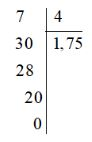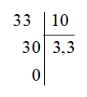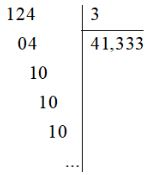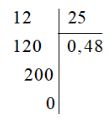## SBT Solution Lesson 1: Irrational numbers. Arithmetic Square Root (C2 Math 7 Horizon) – Math Book

SBT Solution Lesson 1: Irrational numbers. Arithmetic Square Root (C2 Math 7 Horizon)
———–

### Solve problem 1 page 35 SBT Math 7 Creative horizon episode 1

a) Express the following rational numbers as decimals.

$$– \dfrac{7}{4}$$;$$\dfrac{{33}}{{10}}$$;$$\dfrac{{ – 124}}{3}$$;$$\ dfrac{{12}}{{25}}$$

b) In the decimal numbers above, indicate the infinite recurring decimals.

Solution method

Perform division.

Detailed explanation

a) +) Setting the calculation, we get:So $$– \frac{7}{4} = – 1.75.$$

+) Set the calculation, we get:So $$\frac{{33}}{{10}} = 3.3.$$

+) Set the calculation, we get:So $$– \frac{{124}}{3} = \;9{\rm{ }}–{\rm{ }}41,333…{\rm{ }} = –{\rm{ }}41,\ left( 3 \right).$$

Calculating, we get:So $$\frac{{12}}{{25}} = 0.48.$$

b) Among the above decimals, the infinite recurring decimal is – 41, 333… .

### Solution 2 page 35 SBT Math 7 Creative horizon episode 1

Express the following decimal numbers as rational numbers: 7.2; 0.25; 7,(2)

Solution method

+ With finite decimals: Write decimals into decimal fractions

+ With infinite recurring decimals: Separate the integer part and the decimal part and return it to the form of a fraction

Detailed explanation

$$\begin{array}{l}7.2 = \dfrac{{72}}{{10}}=\dfrac{36}{5}\\0.25 = \dfrac{{25}}{{ 100}} = \dfrac{1}{4}\\7,(2) = 7 + 0,(2) = 7 + 2.0,(1) = 7 + 2.\dfrac{1}{9} = \ dfrac{{63}}{9} + \dfrac{2}{9} = \dfrac{{65}}{9}\end{array}$$

### Solution 3 page 35 SBT Math 7 Creative horizon episode 1

Choose the correct statement from the following statements:

a) $$\sqrt 3$$ I

b) $$\sqrt {25}$$ I

c) $$-\pi \in I$$

d) $$\sqrt {\dfrac{{100}}{{47}}}$$ Q

Solution method

We use the definition of sets of numbers $$\mathbb{Q}$$ and $$\mathbb{I}$$

Detailed explanation

a) We have: $$\sqrt 3$$≈1,732050808… so $$\sqrt 3$$ is represented as an infinite non-periodic decimal. It follows that $$\sqrt 3$$ is an irrational number or $$\sqrt 3$$∈ I. Hence a) is true.

b) We have 52 = 25 and 5 > 0 so $$\sqrt {25}$$=5. It follows that $$\sqrt {25}$$ is a rational number, and a rational number is not an irrational number, so $$\sqrt {25}$$∉ I. Hence b) is false.

c) We have: $$-\pi$$ ≈ -3.141592654… so $$-\pi$$ is represented as an infinite non-periodic decimal. Infer that $$-\pi$$ is an irrational number or $$-\pi \in I$$. Hence c) is true.

d) We have: $$\sqrt {\dfrac{{100}}{{47}}}$$ ≈1,458649915… so $$\sqrt {\dfrac{{100}}{{47}}} \ ) is represented as a non-repeating infinite decimal. It follows that \(\sqrt {\dfrac{{100}}{{47}}}$$ is an irrational number, and an irrational number is not a rational number. Hence d) is false.

So the correct statements are a and c.

### Solution 4 page 35 SBT Math 7 Creative horizon episode 1

Calculate:

a) $$– \sqrt {81}$$

b) $$\sqrt {225}$$

c) $$\sqrt {\dfrac{{64}}{{25}}}$$

d) $$\sqrt {{{( – 11)}^2}}$$

e) $$\sqrt {{{(13)}^2}}$$

Solution method

We use the definition of square root: The arithmetic square root of a number $$a$$ is a nonnegative number $$x$$ such that $$x^2 = a$$

Detailed explanation

a) We have 92 = 81 (9 > 0) so $$\sqrt {81} =9$$. Therefore, $$– \sqrt {81}=−9$$

b) We have: 152 = 225 (15 > 0) so $$\sqrt {225} = 15$$

c) We have: $${\left( {\dfrac{8}{5}} \right)^2} = \dfrac{8}{5}.\dfrac{8}{5} = \dfrac{{ 64}}{{25}}$$ so $$\sqrt {\dfrac{{64}}{{25}}} = \dfrac{8}{5}$$

d) We have 112 = (-11)2 (11 > 0) so $$\sqrt {{{( – 11)}^2}} = 11$$

e) We have 13 > 0 so $$\sqrt {{{(13)}^2}} = 13$$

### Solve problems 5 pages 35 SBT Math 7 Creative horizon episode 1

Please replace the sign ? with the appropriate numbers:

 a 256 ? 36 ? $$\sqrt a$$ ? 7 ? 20

Solution method

We use the definition of the square root to find the appropriate number

Detailed explanation

We have:

162 = 256 (16 > 0) so $$\sqrt {256}$$=16 . Hence $$\sqrt a$$ = 16.

72 = 49 so a = 49.

62 = 36 (6 > 0) so $$\sqrt {36}$$=6 . Hence $$\sqrt a$$ = 6.

202 = 400 so a = 400.

Then we fill in the table, we get:

 a 256 49 36 400 $$\sqrt a$$ 16 7 6 20

### Solution 6 page 36 SBT Math 7 Creative horizon episode 1

Use a calculator to calculate the following square roots (round to 3 decimal places).

$$\begin{array}{l}a)\sqrt {133} \\b)\sqrt {99} \\c)\sqrt 7 \\d)\sqrt {1000} \end{array}$$

Solution method

Use a calculator to do calculations

Detailed explanation

$$\begin{array}{l}a)\sqrt {133} \approx 11,533\\b)\sqrt {99} \approx 9,950\\c)\sqrt 7 \approx 2,646\\d)\sqrt {1000 } \approx 31,623\end{array}$$

### Solution 7 page 36 SBT Math 7 Creative horizon episode 1

Uncle Tam hires a florist for a square yard for a total of 36,720 000 VND. Indicate the cost for 1 m2 (including workers and materials) is 255,000 VND. Calculate the length of each side of the yard.

Solution method

Since the yard is square, the area will be equal to the square of 1 side of the yard, so we can calculate the area of ​​the yard by the total amount of the yard divided by the amount per square meter and then the area of ​​the yard. Taking the root of that number will get the length of 1 side of the yard.

Detailed explanation

The area of ​​the square yard is:

36 720 000 : 255 000 = 144 (m2).

Since the yard is square, the area of ​​the yard is equal to the square of the side length, so the length of the side of the square is the arithmetic square root of the area.

So the length of each side of the yard is: $$\sqrt {144}$$ = 12 (m).

So the length of each side of the yard is 12 m.

### Solve problem 8 page 36 SBT Math 7 Creative horizon episode 1

Find the radius of a circle whose area is 42.52 m2

Solution method

We use the formula to calculate the area of ​​a circle with radius R as $$S = 3.14.R^2$$

Detailed explanation

Let R be the radius of the circle, then we have the formula: S = 3.14.R2

The area of ​​the circle is 42.52 m .2 should be CHEAP2 = 42.52 : 3.14 ≈ 13.54

⇔ R = $$\sqrt {13.54}$$ ≈ 3.68 (m)

### Solve lesson 9 page 36 SBT Math 7 Creative horizon episode 1

Find the rational numbers in the following numbers:

5.3; $$\sqrt {\dfrac{1}{9}}$$;$$\sqrt {99}$$;2,(11); 0.456; $$\sqrt {1.21}$$

Solution method

We rely on the definition of what is a rational number to find the rational numbers in the problem

Detailed explanation

We have:

5.3 = $$\dfrac{{53}}{{10}}$$ (where 53; 10 ∈ ℤ and 10 ≠ 0) so 5.3 is a rational number.

$${\left( {\dfrac{1}{3}} \right)^2} = \dfrac{1}{9}\left( {\dfrac{1}{3} > 0} \right)\ ) should \(\sqrt {\dfrac{1}{9}} =\dfrac{1}{3}$$ , (where 1; 3 ∈ ℤ and 3 ≠ 0) should $$\sqrt {\dfrac{ 1}{9}}$$ is a rational number.

$$\sqrt {99}$$ ≈ 9,949874371… is a non-recurring infinite decimal so $$\sqrt {99}$$ is an irrational number.

2,(11) = 2.111111… is an infinite repeating decimal with period 11 so 2,(11) is a rational number.

0.456 is a finite decimal number, so it’s a rational number.

I have 1.12 = 1.21 (1.1 > 0) so $$\sqrt {1.21}$$=1.1. Since 1,1 is a finite decimal, it is a rational number.

So the rational numbers in the above numbers are:$$5,3; \sqrt {\dfrac{1}{9}}; 2,(11); 0.456; \sqrt {1.21}$$

### Solve problems 10 pages 36 SBT Math 7 Creative horizon episode 1

Find the irrational number of the following numbers:

$$\sqrt 5$$;$$\sqrt {\dfrac{{25}}{4}}$$;$$\sqrt {\dfrac{{144}}{{49}}}$$

Solution method

An irrational number is a number that can be written as an infinite non-recurring decimal.

A rational number is a number that can be written as $$\dfrac{a}{b}$$ (with $$a,b \in Z; b \ne 0$$)

Detailed explanation

We have: $$\sqrt 5$$ ≈2,236067977… is an infinite non-recurring decimal number, so $$\sqrt 5$$ is an irrational number.

We have : $${\left( {\dfrac{5}{2}} \right)^2} = \dfrac{5}{2}.\dfrac{5}{2} = \dfrac{{25} }{4}\left( {\dfrac{5}{2} > 0} \right)$$so $$\sqrt {\dfrac{{25}}{4}} = \dfrac{5}{2} \Rightarrow – \sqrt {\dfrac{{25}}{4}} = – \dfrac{5}{2}$$.Where $$– \dfrac{5}{2}$$ is a rational number so \ (\sqrt {\dfrac{{25}}{4}} \) is a rational number

We have: $${\left( {\dfrac{{12}}{7}} \right)^2} = \dfrac{{12}}{7}.\dfrac{{12}}{7} = \dfrac{{144}}{{49}}\left( {\dfrac{{12}}{7} > 0} \right)$$ so $$\sqrt {\dfrac{{144}}{{49 }}} = \dfrac{{12}}{7}$$ . Where $$\dfrac{{12}}{7}$$ is a rational number. Therefore $$\sqrt {\dfrac{{144}}{{49}}}$$ is a rational number.

### Solution 11 page 36 SBT Math 7 Creative horizon episode 1

It is proved that:

– If a fraction has a positive denominator and a denominator has no prime divisors other than 2 and 5, then the fraction is written as a finite decimal.

– If a fraction has a positive denominator and a prime divisor other than 2 and 5, then the fraction is written as a recurring infinite decimal.

Find the infinite recurring decimal in the following rational numbers: $$\dfrac{7}{{20}}$$;$$\dfrac{{25}}{6}$$

Solution method

We divide the numerator by the denominator and find the infinite number of decimals in the given rational numbers

Detailed explanation

Considering the fraction $$\dfrac{7}{{20}}$$ has been simplified, we have the denominator of the fraction is 20 = 22.5 has a prime divisor of 2 and 5, so the fraction is written as a finite decimal.

Considering the fraction $$\dfrac{{25}}{6}$$ has been simplified, we have the denominator of the fraction 6 = 2.3 whose prime divisors are 2 and 3, so this fraction is written as a decimal number. infinitely cyclical stools.

So the recurring decimal is $$\dfrac{{25}}{6}$$